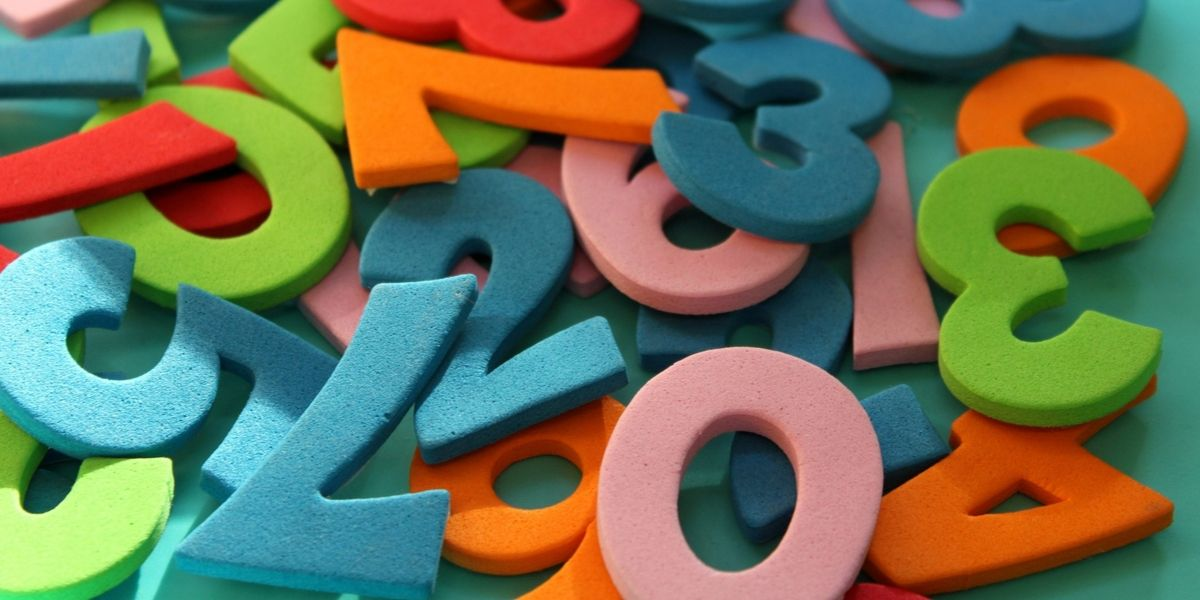October 15, 2019 / by ntweedle

### Coping with Maths in A-level BiologyA common concern among students is coping with maths in A-level Biology. 10% of your A-level Biology marks will require using mathematical skills. If that fills you with dread, do not panic! You don’t need to be studying A-level Maths but a good grounding of GCSE maths is necessary.

##### Coping Tactics

Some of the best tactics to cope with the maths in your Biology exam are:

1. Ensure you have a scientific calculator that you know how to use!
2. Leave the Maths questions until you’ve completed everything else on your Biology exam. It is very easy to get drawn into spending many minutes interpreting a maths question and it may not be the best use of your time!
3. Simplify every question by clearly noting what you are being told and what you are being asked to calculate; then try & find a route through!
4. Practise as many questions as possible from my topic question packs, past papers and the ‘Essential Maths Skills for A-level Biology’ book. It won’t make you perfect but it will give you a much clearer indication of what’s expected and how to manipulate the data.
5. Ensure you can convert units readily e.g. from millimetres to micrometres or grams to kilograms; this really should become second nature.
6. Microscopy calculations-ALWAYS measure in mm and carry out the conversion first. If there is a scale bar shown, you are likely to need to use it. Remember I/AxM.
7. Learn how to calculate percentage change, ratios, powers and standard form – these are calculations you can wrote learn the technique, so you ensure full marks in the exam.
8. Learn formulas such as how to calculate the area and volume of cones and spheres, alongside Hardy Weinberg (yes algebra is back to haunt you!) and Index of Diversity.
9. Graphing and data questions have increased dramatically, with many application questions requiring evidence and quoting data to back up your conclusions. Examiners reports frequently state that students fail to use appropriate data to back up conclusions-so ensure you don’t make that error. Look out for Standard deviation and error bars which can be utilised. If there is a graph or set of data provided you MUST use it!
10. Using statistical tests creates problems for many A-level Biologists but the important thing is you know how to interpret results for tests such as Chi-squared and Spearman’s rank. Depending on your exam board you may have to calculate one statistical test but the formula would be provided. More commonly you have to interpret the answer using degrees of freedom and the critical value including reference to the terms probability and chance.

Nicky Tweedle 30/9/2019